# Trigonometry Common Values

GCSELevel 4-5AQAEdexcelOCRWJEC

## Trigonometry Common Values

Up to this point, we’ve let our calculators do the heavy lifting when it comes to evaluating the $3$ trigonometry functions, but there are some values of the trigonometry functions that you have to remember.

Make sure you are happy with the following topics before continuing:

Level 4-5GCSEAQAEdexcelOCRWJEC

## Values You Need to Learn

Specifically, you will have to remember:

$\sin$, $\cos$, and $\tan$ of $0\degree, 30\degree, 45\degree, 60\degree,$ and $90\degree$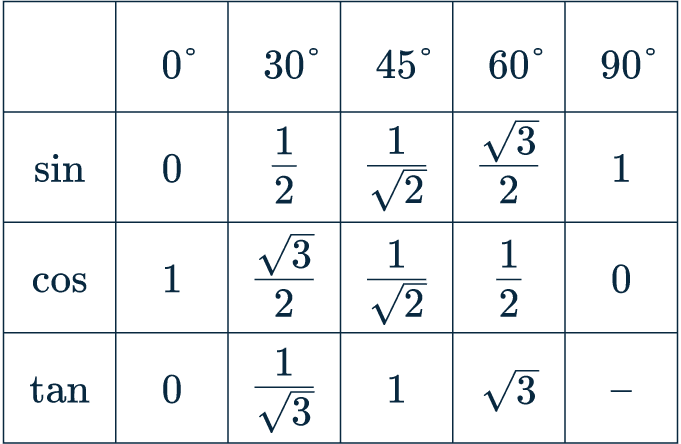You need to remember all of these values.

Level 4-5GCSEAQAEdexcelOCRWJEC

## Trigonometric Triangles

Remembering the values in the table may be a little tricky if you don’t have a photographic memory so you might find it easier to remember two important triangles:

1. Equilateral Triangle (cut in half)
2. Right Angle Isosceles Triangle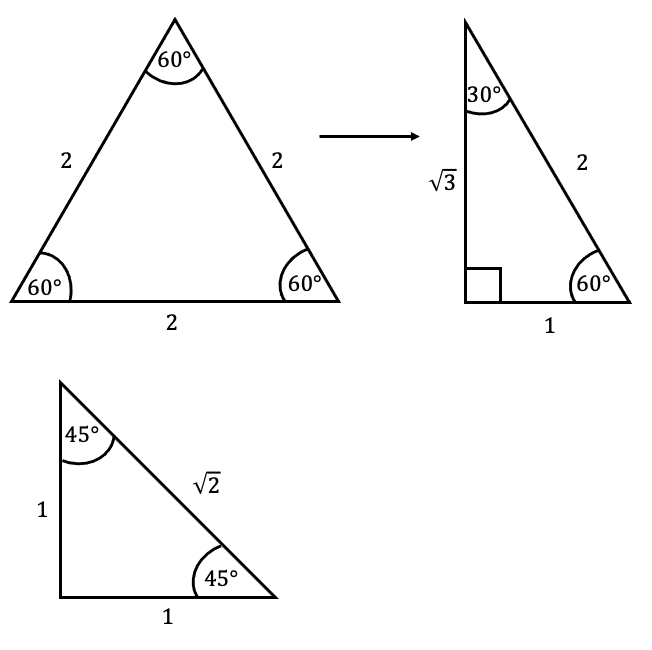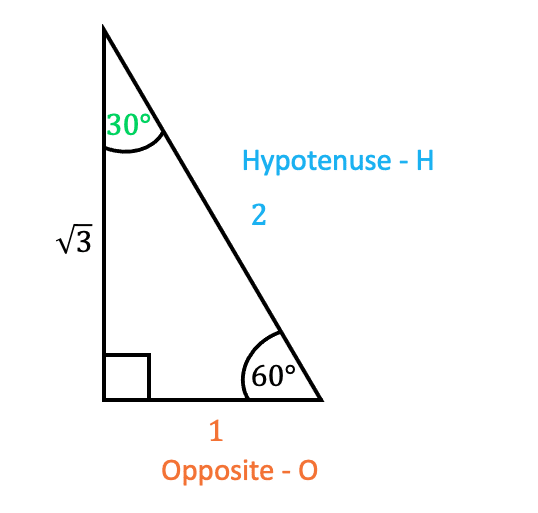To use these triangles, we use SOH-CAH-TOA rules to calculate common trigonometry values.

For instance, say we wanted to know $\sin{30}$, we use the rule

$\sin{\textcolor{#00d865}{x}}=\dfrac{\textcolor{#f95d27}{O}}{\textcolor{#10a6f3}{H}}$

and take the corresponding values from the triangle that we need.

$\sin{\textcolor{#00d865}{30}}=\dfrac{\textcolor{#f95d27}{1}}{\textcolor{#10a6f3}{2}}$

Level 4-5GCSEAQAEdexcelOCRWJEC

## Example

Find the value of $y$ shown on the right-angled triangle.

[2 marks]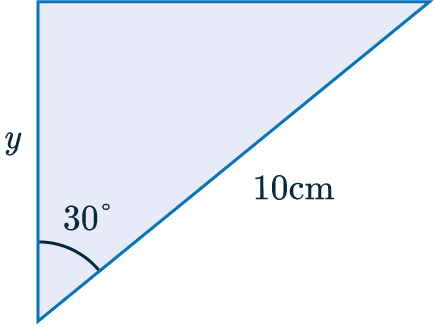First we have to find which equation we need to use.

The hypotenuse $=\textcolor{blue}{10}$ cm

The adjacent $= \textcolor{limegreen}{y}$

This means we use $\textcolor{purple}{C}\textcolor{limegreen}{A}\textcolor{blue}{H}$

$\textcolor{purple}{\cos(30\degree)} = \dfrac{\textcolor{limegreen}{y}}{\textcolor{blue}{10}}$

We can see in the table that $\textcolor{purple}{\cos(30\degree)} = \textcolor{purple}{\dfrac{\sqrt{3}}{2}}$, putting this into the equation we can solve for $\textcolor{limegreen}{y}$.

$\textcolor{purple}{\dfrac{\sqrt{3}}{2}} = \dfrac{\textcolor{limegreen}{y}}{\textcolor{blue}{10}}$

$\dfrac{\textcolor{blue}{10}\textcolor{purple}{\sqrt{3}}}{\textcolor{purple}{2}} = \textcolor{limegreen}{y}$

$5\sqrt{3} = \textcolor{limegreen}{y}$

Level 4-5GCSEAQAEdexcelOCRWJEC

## Trigonometry Common Values Example Questions

Each term is one of a small list of common trigonometry values that students are required to remember. Here,

$\sin(60\degree)=\dfrac{\sqrt{3}}{2}$

as well as,

$\cos(30\degree)=\dfrac{\sqrt{3}}{2}$

Thus the calculation is,

$\sin(60\degree)+ \cos(30\degree)=\dfrac{\sqrt{3}}{2}+\dfrac{\sqrt{3}}{2}=\sqrt{3}$Gold Standard Education

Each term is one of a small list of common trigonometry values that students are required to remember:

$\tan(45\degree)=1$

$\sin(30\degree)=\dfrac{1}{2}$

and

$\tan(60\degree)=\sqrt{3}$

Thus the calculation is,

$\dfrac{\tan(45\degree)}{\sin(30\degree)} \times 10\tan(60\degree)=\dfrac{1}{\frac{1}{2}} \times 10(\sqrt{3})=2\times10\sqrt{3}=20\sqrt{3}$Gold Standard Education

Each term is one of a small list of common trigonometry values that students are required to remember:

$\tan(45\degree)=1$

$\cos(30\degree)=\dfrac{\sqrt{3}}{2}$

and

$\cos(60\degree)=\dfrac{1}{2}$

Thus the calculation is,

$\dfrac{\tan(45\degree)+\cos{(30\degree)}}{\tan(45\degree)} \times \cos(60\degree)=\dfrac{1+\frac{\sqrt{3}}{2}}{1} \times \dfrac{1}{2}=\dfrac{2+\sqrt{3}}{2}\times \dfrac{1}{2}=\dfrac{2+\sqrt{3}}{4}$Gold Standard Education

This question requires the use of Pythagoras. We are given the hypotenuse and want the find the opposite side length, hence,

$\sin(30\degree)=\dfrac{x}{16}$

Thus to find $x$ we simply multiply both sides by 16 and use the common trigonometry value of $\sin(30\degree) =\dfrac{1}{2}$

$x=16\times\sin(30\degree)=16\times \dfrac{1}{2}=8$ cm.Gold Standard Education

This question requires the use of Pythagoras. We are given the adjacent length and want the find the opposite side length, hence,

$\tan(30\degree)=\dfrac{x}{12}$

Thus to find $x$ we multiply both sides by $12$ and use the common trigonometry value of $\tan(30\degree) =\dfrac{1}{\sqrt{3}}$

$x=12\times\tan(30\degree)=12\times \dfrac{1}{\sqrt{3}}=12\times \dfrac{\sqrt{3}}{3}=4\sqrt{3}$ cm.Gold Standard Education

## Trigonometry Common Values Worksheet and Example Questions

### (NEW) Trig Common Values Exam Style Questions - MME

Level 4-5NewOfficial MME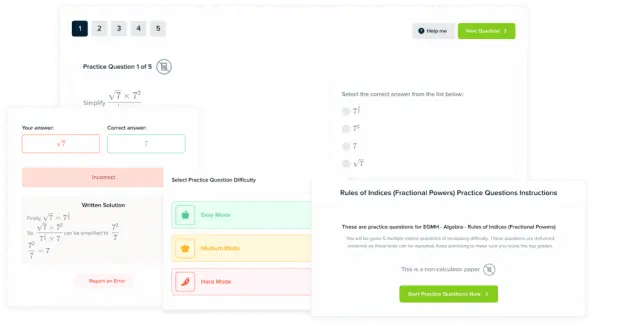Product